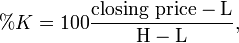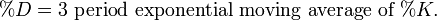# Stochastics

The Stochastic Oscillator was developed by Dr. George Lane to track market momentum. It is an indicator that measures the relationship between an issue’s closing price and its price range over a predetermined period of time.The following equation is given only to highlight how the % figure is calculated. The paragraph under the formula gives a simple, succinct explanation Stochastics.

This method attempts to predict price turning points by comparing the closing price of a security to its price range.

The indicator is defined as follows:where H and L are respectively the highest and the lowest price over the lastperiods, andThe calculation above finds the range between an asset’s high and low price during a given period of time. The current securities price is then expressed as a percentage of this range with 0% indicating the bottom of the range and 100% indicating the upper limits of the range over the time period covered. The idea behind this indicator is that prices tend to close near the extremes of the recent range before turning points.